## Course Description -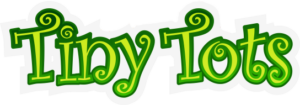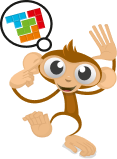## The Aloha Programme

ALOHA is a mental development programme designed specifically for children ages 5 to 13. The program uses the abacus as a key instrument for teaching Mental Arithmetic, enabling the development of the right hemisphere of the brain, a key instrument for the teaching of Mental Arithmetic. In this hemisphere resides the ability of visual memory, concentration and orientation, control of nonverbal aspects of communication, and intuition.ALOHA Mental Arithmetic classes are available ONLINE (all levels) or physical in Ongard Comunity Centre, Dublin 15 only for Level 1 students## Course InformationTots
Junior Cycle

Levels
1 – 4

Tots
Pro Cycle

Levels
5 – 8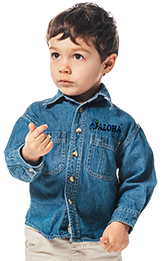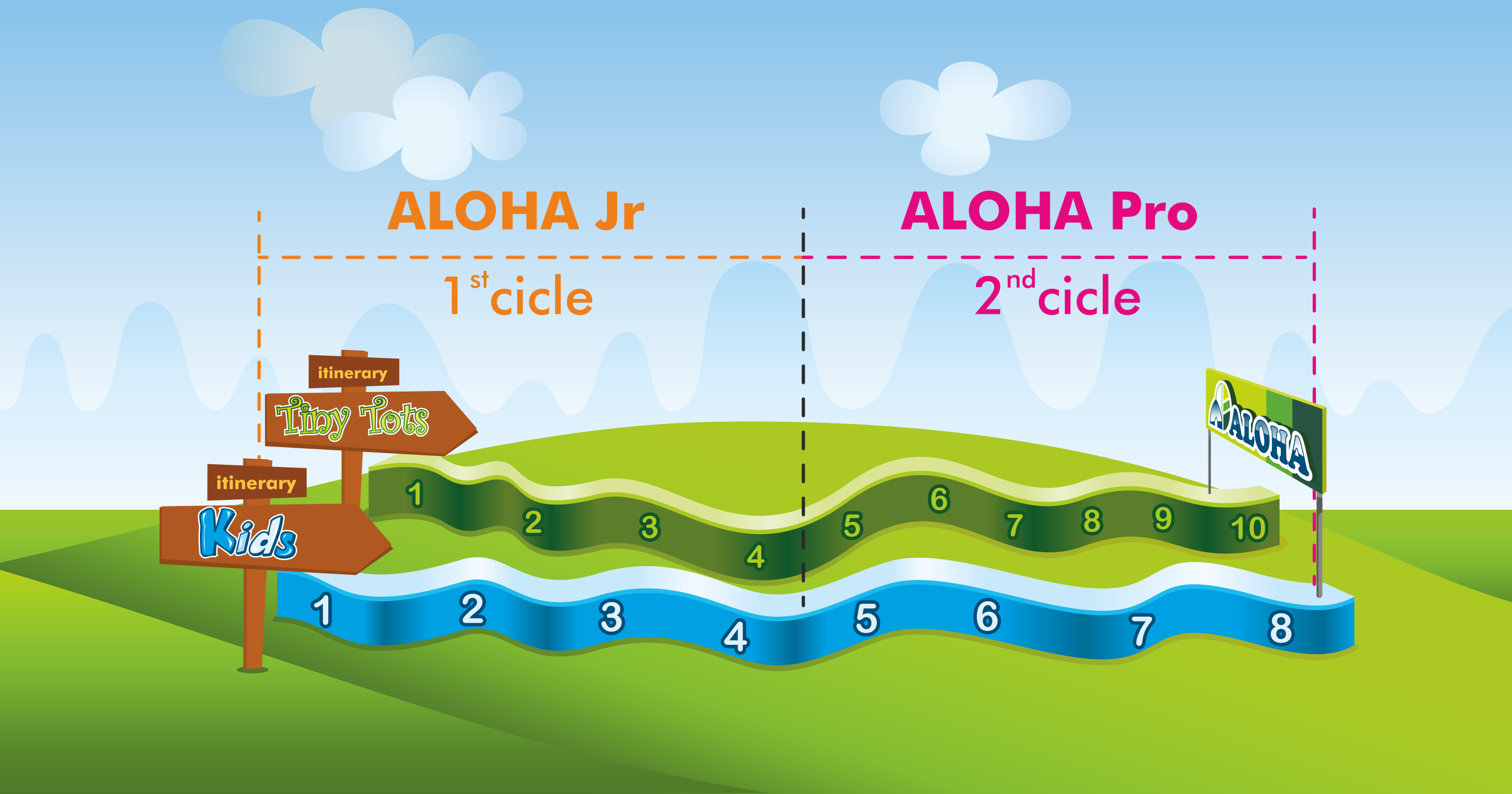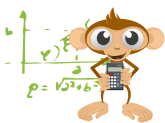## Class Structure

Initial Games

ALOHA Games

Brain Gym ( online classes)

10

Mental Development

Speed Test

Number Dictation

Flashcards

10

Arithmetic’s

Oral review,

Book A,

Book review A and B

Orals of the day

30

Break

Break

10

Worksheets and Mental Arithmetic

Weekly Worksheets

Mental Calculations

40

Final Games

ALOHA Challenge

Kahoot / Games

10

110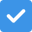A minimum of
60%
is required to successfully
pass the level.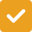Assessments will be
carried out in centralized
assessment locations
twice per year in January
and June, or online during
the pandemic restrictions.On successful completion
of the
Assessment students is
issued with a Level
Diploma in a 30 working
days period after
completion of assessment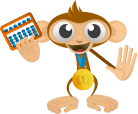## Class Content per Level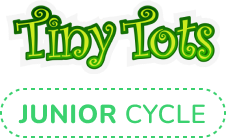## Level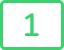#### Tiny Tots

Level 1 Content

• Introduction to Abacus usage
• Introduction to Mental Theory
• Addition and subtraction from 1 to 9

EX: 2 + 5 + 2 -7 = ?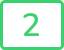#### Tiny Tots

Level 2 NEW Content

• Addition and subtraction from 1 to 99

EX: 38 + 51 – 78 = ?

NEW
Content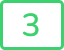#### Tiny Tots

Level 3 NEW Content

• Introduction to the multiplication tables: tables 1 to 5
NEW
Content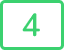#### Tiny Tots

Level 4 NEW Content

• 2 x 1 and 3 x 1 digit multiplications:
•  tables 1 to 5

EX: 643 x 3 = ?

• Introduction to tables from 6 to 9
• 2 + 1 digit divisions

EX: 27 ÷3 = ?

NEW
Content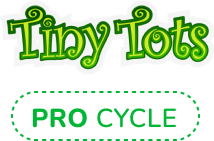## Level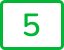#### Tiny Tots

Level 5 NEW Content

• 2 and 3 digit addition and subtraction

Ex: 563 – 421 + 314 = ?

• 3 x 1 digit multiplication:
• tables 1 to 9

Ex: 882 x 6 = ?

• Divisions of 3÷1 digits

Ex: 847 ÷ 7 = ?

NEW
Content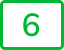#### Tiny Tots

Level 6 NEW Content

• 4 x 1 digit multiplication

EX: 6696 x 4 = ?

• 4 / 1 digit division

EX: 3486 ÷ 3 = ?

NEW
Content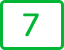#### Tiny Tots

Level 7 NEW Content

• 4 digit addition and subtraction

EX: 8325 – 2537 + 6278 = ?

•  3 digit decimals

EX: 34,8 + 16,6 = ?

• 2 x 2 digit multiplication

EX: 93 x 65 = ?

• 3 ÷ 2 digit divisions

EX. 392÷ 98 = ?

NEW
Content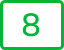#### Tiny Tots

Level 8 NEW Content

• 3 x 2 digit multiplication

EX.: 924 x 86 = ?

EX: 4/15 + 7/15= ?

NEW
Content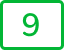#### Tiny Tots

Level 8 NEW Content

• 4 digit decimals

Ex. 46,27 + 78,78 = ?

• 4 x 2 digit multiplication

Ex: 3946 x 46 = ?

• 4÷2 and 5÷2 digit divisions

Ex: 83312 ÷ 72 = ?

• Fractions multiplication and division

Ex: 23/48 ÷ 69/92 = ?

• Powers

Ex: 52² = ?

NEW
Content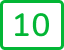#### Tiny Tots

Level 10 NEW Content

• 5 digit addition and subtraction

Ex: 56629 + 500 – 4488 = ?

• 5 digit decimal

Ex: 393,47 + 41,29 – 42,91 = ?

• 4 digit Square roots

Ex: √8836 = ?

• Percentages

Ex. 33% of 500 = ?

• Combinations of arithmetic operations

Ex: 9 x (56 + 31 – 17) ÷ 7 = ?

NEW
Content